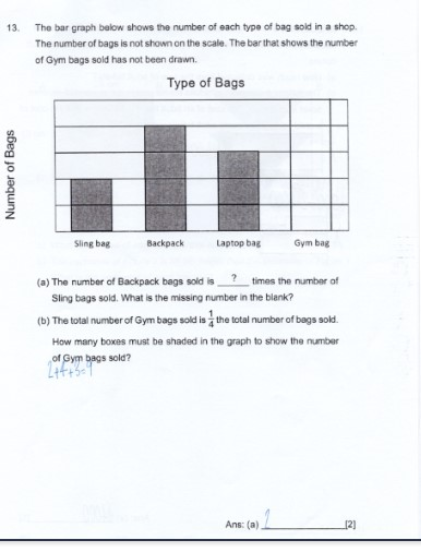# QuestionHow to tackle this question?

(a)  If each unit is u, backpack is 4u, sling bag is 2u, so 4u/2u = 2

Backpack is 2 times of sling bags.

(b) let the unknown gym bags be p.   Total of the other 3 bags type = 2 + 4 +3 = 9

p / (9+p) = 1/4

4p = 9+p => 3p =9 => p = 3

Hence 3 boxes must be shaded for gym bags.

0 Replies 1 Like